# bzoj2648 SJY摆棋子（不带修改的KDtree的学习）

Description

Input

Output

Sample Input
2 3
1 1
2 3
2 1 2
1 3 3
2 4 2

Sample Output
1
2

KD tree
http://blog.csdn.net/silangquan/article/details/41483689

http://blog.sina.com.cn/s/blog_8d5d2f040101888r.html

（不是说好只有M+1行的吗）

kdtree的原理想必都明白吧
（看看那些大佬的blog就好了，这里不再废话）

(d,d) 该节点代表的子树的根节点
(l,r) 该节点的左右儿子
(mx,mx) 该节点代表的子树管理的区间右上角
(mn,mn) 该节点代表的子树管理的区间左下角

kdtree的构建和插入是不一样的
(可能有人会说，肯定不一样啊干，

# 一 . kd的构建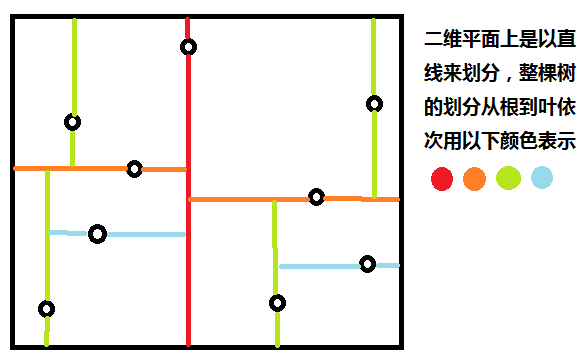nth_element函数

# 不要忘了build返回值是int，我们要靠build找到这棵树的根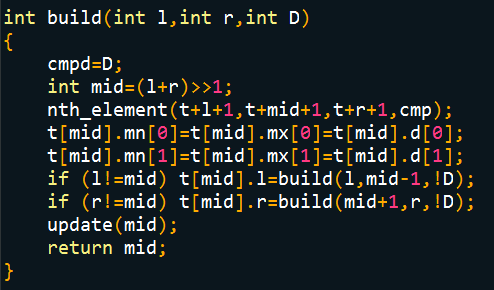# 二 . update和cmp

cmp

update

（担心有人不懂，来看一下图）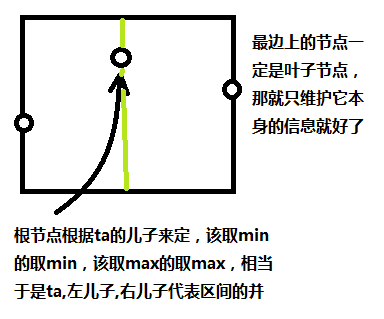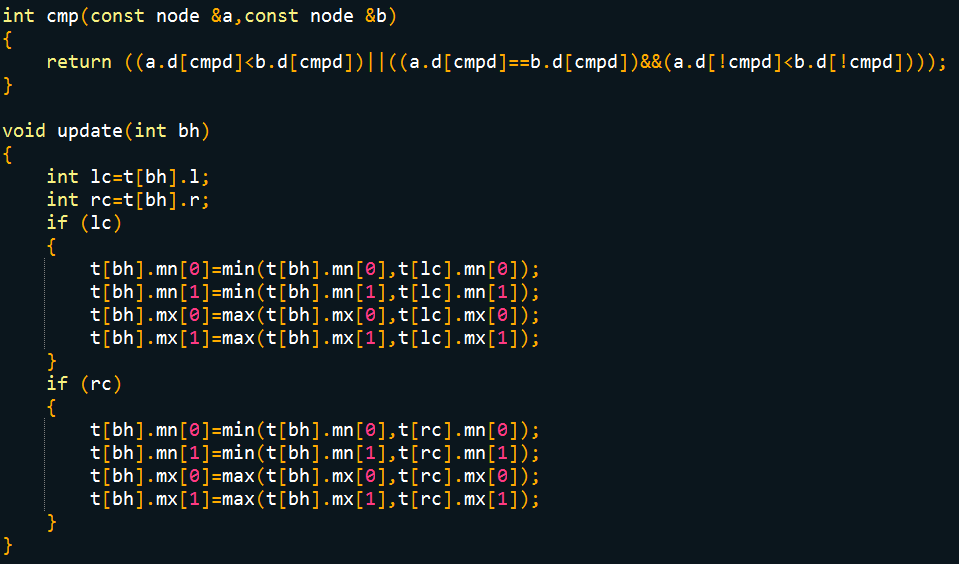insert

# 注意，在该坐标相等的情况下归入右节点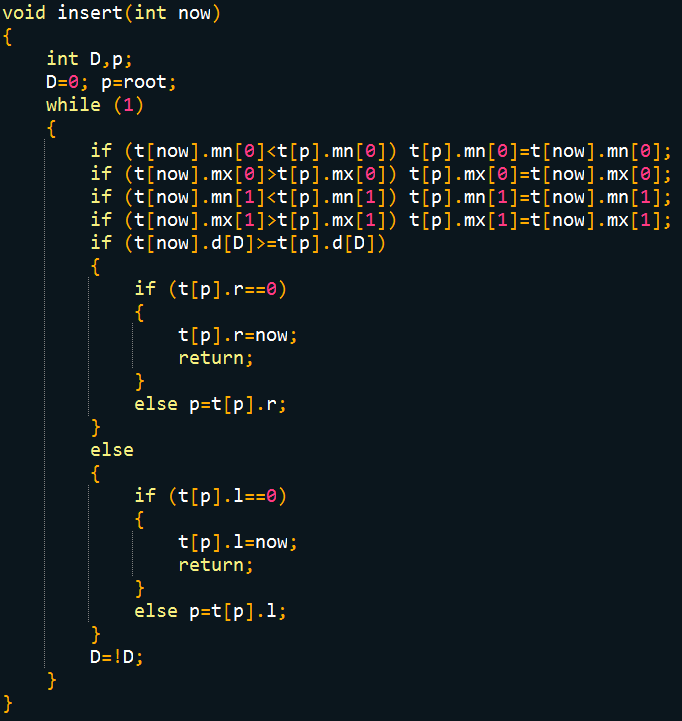# 四 . kdtree的查询

d0表示当前点到查询点的距离
dl表示当前点左儿子到查询点的距离
dr表示当前点右儿子到查询点的距离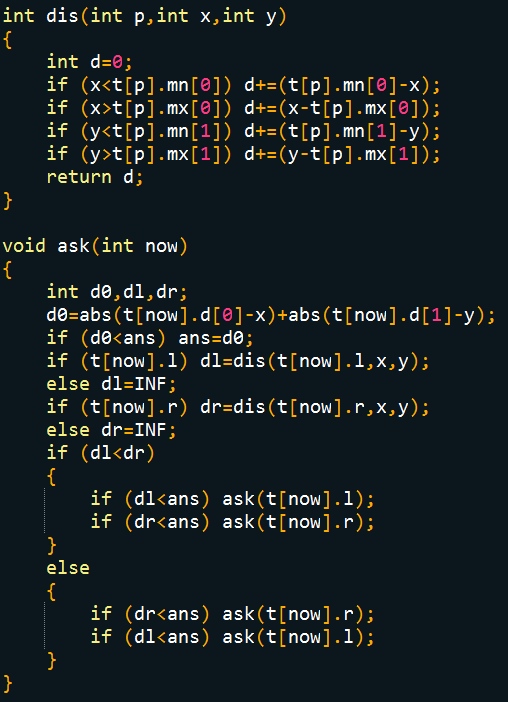这里写代码片
#include<cstdio>
#include<cstring>
#include<iostream>
#include<algorithm>

using namespace std;

const int INF=0x33333333;
struct node{
int d,l,r,mn,mx;
};
node t;
int n,m,x,y,opt,ans,cmpd,root;

int cmp(const node &a,const node &b)
{
return ((a.d[cmpd]<b.d[cmpd])||((a.d[cmpd]==b.d[cmpd])&&(a.d[!cmpd]<b.d[!cmpd])));
}

void update(int bh)
{
int lc=t[bh].l;
int rc=t[bh].r;
if (lc)
{
t[bh].mn=min(t[bh].mn,t[lc].mn);
t[bh].mn=min(t[bh].mn,t[lc].mn);
t[bh].mx=max(t[bh].mx,t[lc].mx);
t[bh].mx=max(t[bh].mx,t[lc].mx);
}
if (rc)
{
t[bh].mn=min(t[bh].mn,t[rc].mn);
t[bh].mn=min(t[bh].mn,t[rc].mn);
t[bh].mx=max(t[bh].mx,t[rc].mx);
t[bh].mx=max(t[bh].mx,t[rc].mx);
}
}

int build(int l,int r,int D)
{
cmpd=D;
int mid=(l+r)>>1;
nth_element(t+l+1,t+mid+1,t+r+1,cmp);
t[mid].mn=t[mid].mx=t[mid].d;
t[mid].mn=t[mid].mx=t[mid].d;
if (l!=mid) t[mid].l=build(l,mid-1,!D);
if (r!=mid) t[mid].r=build(mid+1,r,!D);
update(mid);
return mid;
}

void insert(int now)
{
int D,p;
D=0; p=root;
while (1)
{
if (t[now].mn<t[p].mn) t[p].mn=t[now].mn;
if (t[now].mx>t[p].mx) t[p].mx=t[now].mx;
if (t[now].mn<t[p].mn) t[p].mn=t[now].mn;
if (t[now].mx>t[p].mx) t[p].mx=t[now].mx;
if (t[now].d[D]>=t[p].d[D])
{
if (t[p].r==0)
{
t[p].r=now;
return;
}
else p=t[p].r;
}
else
{
if (t[p].l==0)
{
t[p].l=now;
return;
}
else p=t[p].l;
}
D=!D;
}
}

int dis(int p,int x,int y)
{
int d=0;
if (x<t[p].mn) d+=(t[p].mn-x);
if (x>t[p].mx) d+=(x-t[p].mx);
if (y<t[p].mn) d+=(t[p].mn-y);
if (y>t[p].mx) d+=(y-t[p].mx);
return d;
}

{
int d0,dl,dr;
d0=abs(t[now].d-x)+abs(t[now].d-y);
if (d0<ans) ans=d0;
if (t[now].l) dl=dis(t[now].l,x,y);
else dl=INF;
if (t[now].r) dr=dis(t[now].r,x,y);
else dr=INF;
if (dl<dr)
{
}
else
{
}
}

int main()
{
scanf("%d%d",&n,&m);
for (int i=1;i<=n;i++)
scanf("%d%d",&t[i].d,&t[i].d);
root=build(1,n,0);
for (int i=1;i<=m;i++)
{
scanf("%d%d%d",&opt,&x,&y);
if (opt==1)
{
n++;
t[n].mn=t[n].mx=t[n].d=x;
t[n].mn=t[n].mx=t[n].d=y;
insert(n);
}
else
{
ans=INF;
}